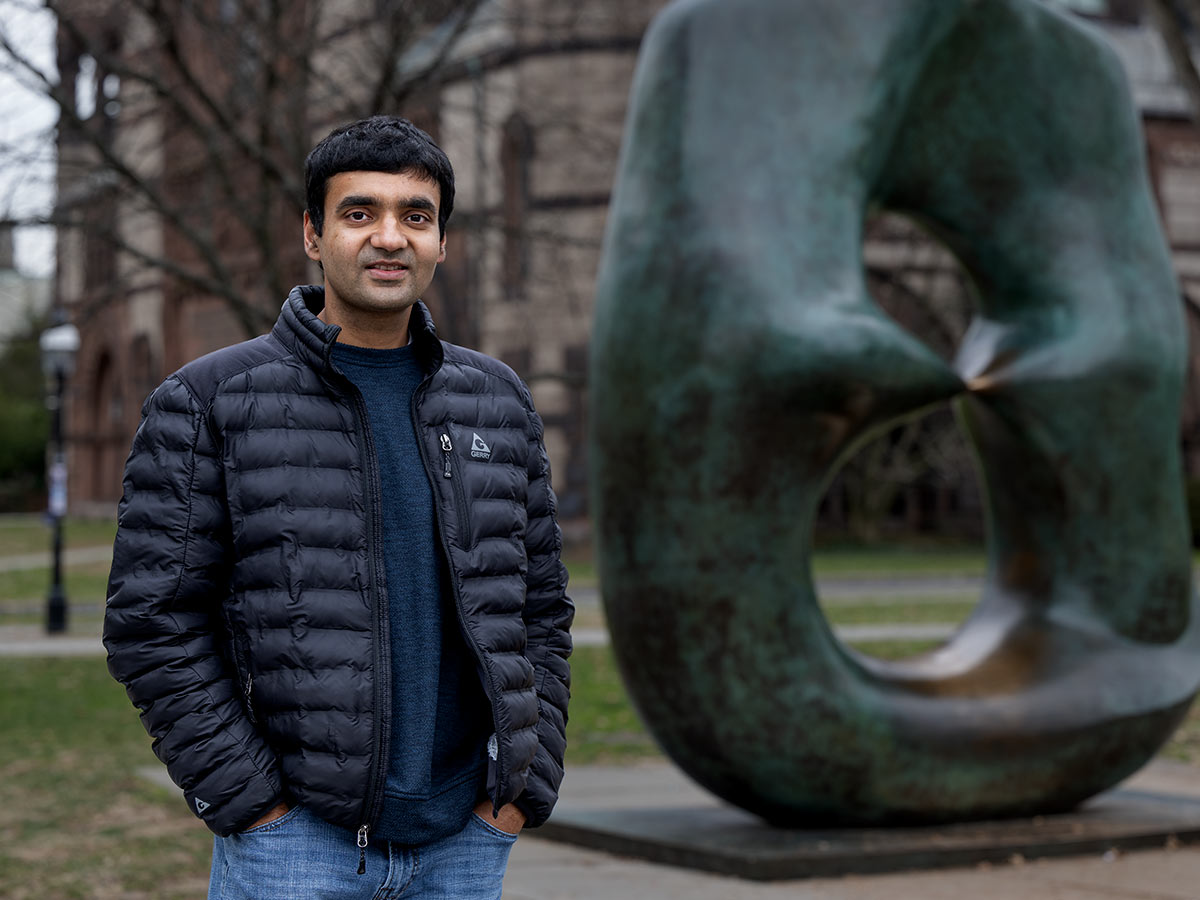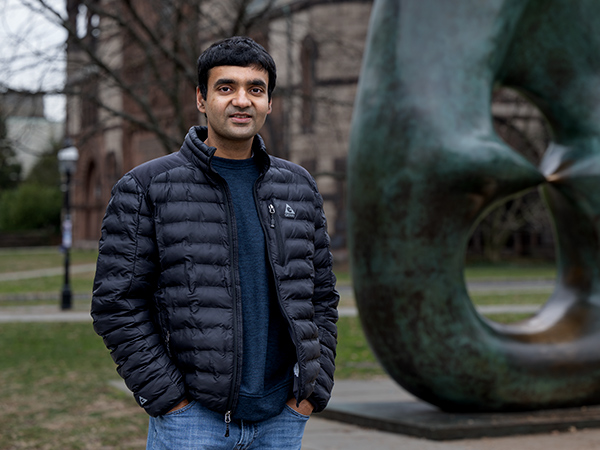### Bhargav Bhatt

Fernholz Joint Professor, Institute for Advanced Study and Princeton University Gehring Professor, University of Michigan

BackThe Infosys Prize 2023 in Mathematical Sciences is awarded to Prof. Bhargav Bhatt for his outstanding contributions to arithmetic geometry and commutative algebra. Bhatt’s fundamental work on prismatic cohomology (joint with Peter Scholze), his work around the direct summand conjecture in commutative algebra, introduces new ideas and powerful methods in an area at the heart of pure mathematics.

### Scope and Impact of Work

Prof. Bhargav Bhatt’s two main research areas are p-adic geometry (in the shape of p-adic Hodge theory) and commutative algebra. Prof. Bhatt has made groundbreaking contributions to both areas and his research has revealed completely unexpected relationships between the two, showing that the deepest questions in both have surprising and very close connections. The theory of prismatic cohomology of Bhatt and Scholze represents a truly fundamental discovery in arithmetic algebraic geometry, which will eventually be part of the standard toolkit of every mathematician working in the area. It has already attracted a huge amount of attention and given rise to many applications. Prof. Bhatt is at the forefront of these developments. He is among the top leaders in arithmetic algebraic geometry worldwide.

Prismatic cohomology is the right theory of p-adic cohomology of p-adic schemes which unifies all the previously existing theories and sheds new light on the structure of those. Much work by mathematicians over the past five decades can now be viewed retrospectively as steps toward the Bhatt-Scholze theory of prismatic cohomology.

### Bio

Prof. Bhargav Bhatt is the Fernholz Joint Professor at the Institute of Advanced Study and Princeton University since 2022. He has been on the faculty at the University of Michigan as Gehring Professor, since 2014. He obtained his Ph.D. from Princeton University in 2010, and his B.S. in Applied Mathematics from Columbia University in 2005. He was born in Mumbai and studied until high school there.

Prof. Bhatt’s work has been recognized by a number of prestigious prizes: Clay Research Award (2021), New Horizons Prize in Mathematics (2021), Nemmers Prize (2022). He was a Plenary speaker at the International Congress of Mathematicians in 2022.

2005-2010

2014

2021

2022

2023

## 2023

2005-2010
2014
2021
2022
2023

### Jury Citation

Prof. Bhargav Bhatt is an internationally renowned expert in arithmetic algebraic geometry and commutative algebra. He has made fundamental contributions to both subjects. Prof. Bhatt is at the forefront of a revolution in p-adic geometry. For each prime p, there is a p-adic geometry, with a very different flavor from the geometry of the real world we live in. Despite this difference, p-adic geometry has found spectacular applications in solving famous conjectures in number theory, like the Mordell conjecture and Fermat’s Last Theorem. One of Bhatt's significant mathematical contributions is his introduction (in joint work with Peter Scholze) of the theory of prismatic cohomology. He has applied these developments to solve long standing problems in commutative algebra.Bhargav Bhatt

I would like to congratulate Bhargav for winning the Infosys Prize 2023 in Mathematical Sciences. His work has produced some of the most exciting and impactful mathematics done anywhere in the world over the last decade. His work on prismatic cohomology has introduced a powerful set of methods in p-adic geometry. And there is so much more to come!

#### Chandrashekhar Khare

Jury Chair
Mathematical Sciences